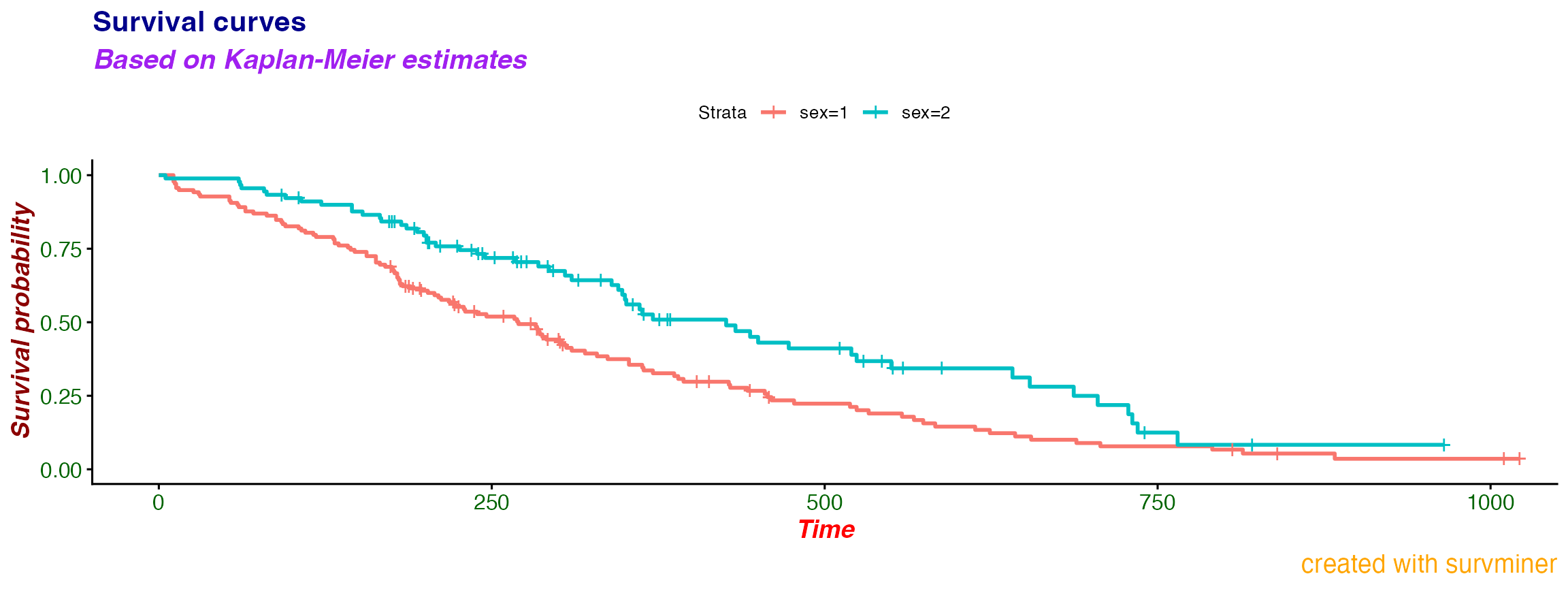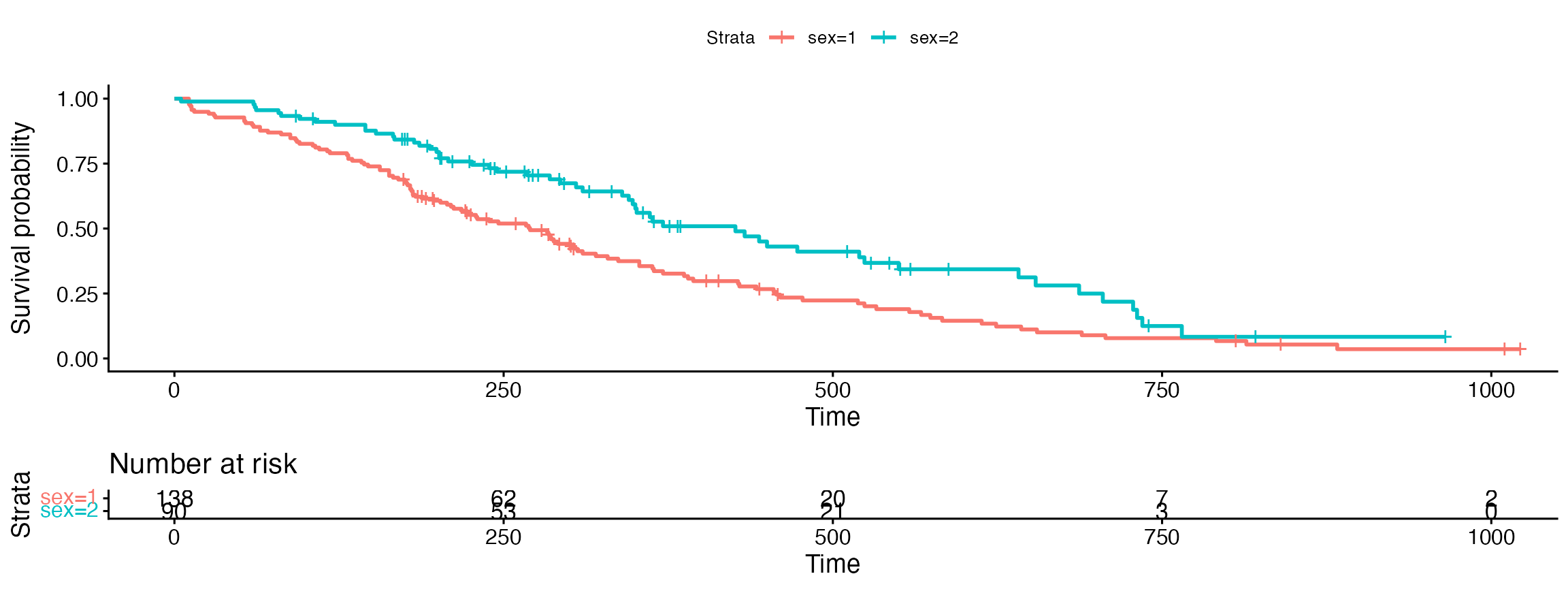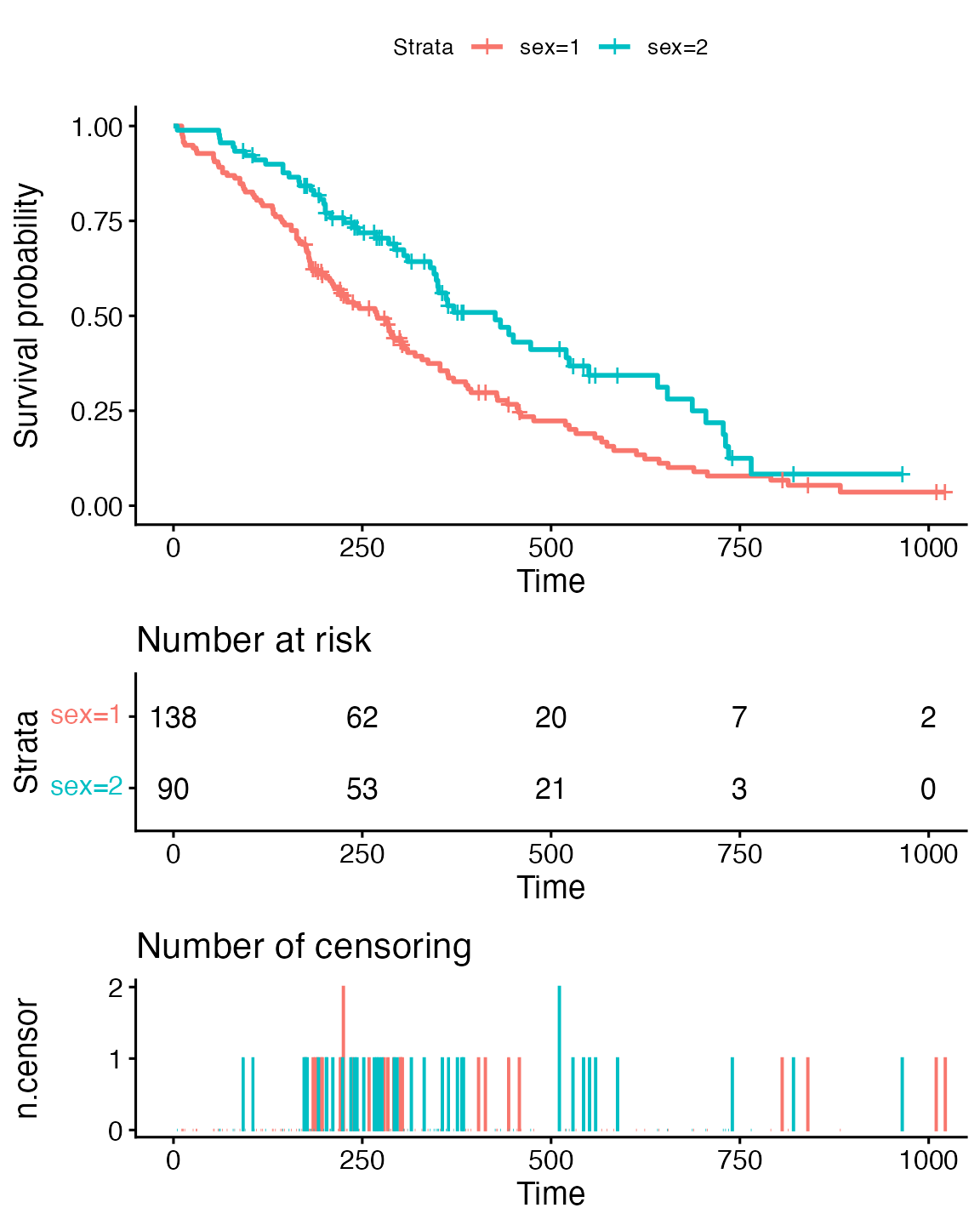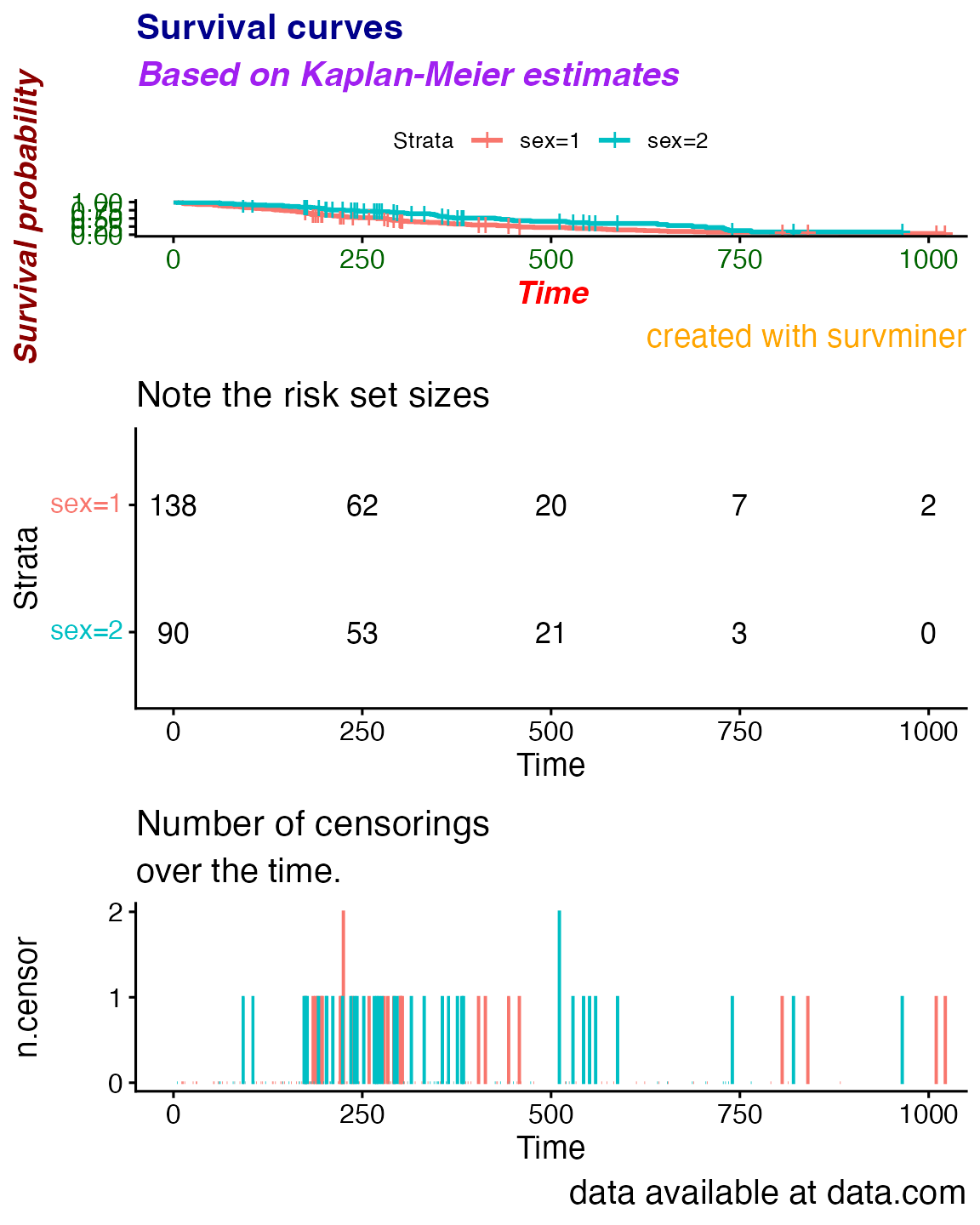library("survminer")

This vignette covers changes between versions 0.2.4 and 0.2.5 for additional texts and fonts customization enabled for subtitles and captions.

# Survival plot

## Basic

Compare the basic plot

library("survival")
fit<- survfit(Surv(time, status) ~ sex, data = lung)

# Drawing survival curves
ggsurvplot(fit, data = lung)## Customized

with the plot where every possible text on a plot is specified

ggsurvplot(fit, data = lung,
title = "Survival curves", subtitle = "Based on Kaplan-Meier estimates",
caption = "created with survminer",
font.title = c(16, "bold", "darkblue"),
font.subtitle = c(15, "bold.italic", "purple"),
font.caption = c(14, "plain", "orange"),
font.x = c(14, "bold.italic", "red"),
font.y = c(14, "bold.italic", "darkred"),
font.tickslab = c(12, "plain", "darkgreen"))# Risk table

Now allow risk.table to be displayed.

## Basic

Please compare basic plot with a risk table

ggsurvplot(fit, data = lung, risk.table = TRUE)## Customized

with the plot where every possible text on a plot and table is specified

ggsurvplot(fit, data = lung,
title = "Survival curves", subtitle = "Based on Kaplan-Meier estimates",
caption = "created with survminer",
font.title = c(16, "bold", "darkblue"),
font.subtitle = c(15, "bold.italic", "purple"),
font.caption = c(14, "plain", "orange"),
font.x = c(14, "bold.italic", "red"),
font.y = c(14, "bold.italic", "darkred"),
font.tickslab = c(12, "plain", "darkgreen"),
########## risk table #########,
risk.table = TRUE,
risk.table.title = "Note the risk set sizes",
risk.table.subtitle = "and remember about censoring.",
risk.table.caption = "source code: website.com",
risk.table.height = 0.45)# ncens plot

Finally, allow ncens.plot to be displayed.

## Basic

Please compare basic plot with a risk table and a ncens plot

ggsurvplot(fit, data = lung, risk.table = TRUE, ncensor.plot = TRUE)## Customized

with the full customization

ggsurvplot(fit, data = lung,
title = "Survival curves", subtitle = "Based on Kaplan-Meier estimates",
caption = "created with survminer",
font.title = c(16, "bold", "darkblue"),
font.subtitle = c(15, "bold.italic", "purple"),
font.caption = c(14, "plain", "orange"),
font.x = c(14, "bold.italic", "red"),
font.y = c(14, "bold.italic", "darkred"),
font.tickslab = c(12, "plain", "darkgreen"),
########## risk table #########,
risk.table = TRUE,
risk.table.title = "Note the risk set sizes",
risk.table.subtitle = "and remember about censoring.",
risk.table.caption = "source code: website.com",
risk.table.height = 0.35,
ncensor.plot = TRUE,
ncensor.plot.title = "Number of censorings",
ncensor.plot.subtitle = "over the time.",
ncensor.plot.caption = "data available at data.com",
ncensor.plot.height = 0.35)